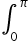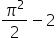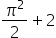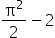Maths-
General
Easy

Question

# If f : R →R; f(x) = sin x + x, then the value of(f-1 (x)) dx, is equal to

##p2None of theseHint:

## The correct answer is:### The summing of discrete data is indicated by the integration. To determine the functions that will characterise the area, displacement, and volume that result from a combination of small data that cannot be measured separately, integrals are calculated.We are aware that differentiation is the process of discovering a function's derivative and integration is the process of discovering a function's antiderivative. Thus, both processes are the antithesis of one another. Therefore, we can say that differentiation is the process of differentiation and integration is the reverse.Here we have given the function f : R →R; f(x) = sin x + xHere we used the concept of integration and the inverse functions to solve the question. Finding an antiderivative of a function is the procedure known as integration. The process of adding the slices to complete it is comparable. The process of integration is the opposite of that of differentiation. So the final answer is.#### With Turito Foundation.#### Get an Expert Advice From Turito.